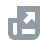Pay with
Markets
NFT
New
English
USDAccount FunctionsTutorialBinance Fan TokenBinance EarnCrypto Deposit/WithdrawalCrypto DerivativesFinanceAPISecurityNFTVIP

# What Are Isolated Margin Trading Positions

2021-09-15 06:38

### .css-1vsinja{box-sizing:border-box;margin:0;min-width:0;color:#000000;}.css-1lohbqv{box-sizing:border-box;margin:0;min-width:0;font-weight:600;}What are Isolated Margin Trading Positions?

Isolated Margin Trading Positions are used to calculate the cost and profit/loss of a position in historical transactions. The isolated margin position is not dependent on the amount of funds in your account or your borrowing behavior. Instead, it uses cumulative data from historical trades of the trading pair (long and short) to calculate. The position information is recalculated and updated every 5 minutes. For example, if you make a margin trade within the 5 minutes before the system recalculates, the position value and profit/loss calculations will be based on the prevailing calculations.

If you open a long/short margin position over a series of transactions, the Isolated Margin trading positions calculation can be used to calculate the average cost of your trades. It is convenient for checking your profit and loss and position value based on your historical trading activity, so you can make better investment decisions.

1. How to calculate isolated margin trading positions/cumulative margin positions?
Trading position refers to the net buy (long) / net sell (short) amount of an asset you traded in a particular isolated trading pair since you opened the initial position. For example, if you opened a margin position over a series of transactions, you can use the cumulative calculation to help determine your net position size.
Suppose you opened a BTCUSDT isolated margin position and made a series of transactions following your initial position. The net purchase quantity after each transaction is as follows:
At T+3, you will have a long position of 1 BTC.
At T+4, you will have a short position of 4 BTC
At T+5, you will have no position.
*Assuming on T+3, you have a long position of 1 BTC and hold 1 BTC in your margin account, you then transferred the 1 BTC to your spot account, i.e. no BTC of assets in your isolated margin account, your trading position will still have a long position of 1 BTC.
2. How to calculate the cost price in isolated margin positions?
For long positions:
Cost Price = ∑ (Buy Quantity * Buy Price) / Position Size (since the initial position was opened)
In this case, any additional long positions following the initial position will be accounted for and recalculated to determine the new cost price.
For short positions:
Cost Price= ∑ (Sell Quantity * Sell Price)/ Position Size (since the initial position was opened)
In this case, any additional short positions following the initial position will be accounted for and recalculated to determine the new cost price.
A weighted average takes into account the quantity and price purchased with each trade. In other words, if you buy an additional 2 BTC, the price you pay will affect the average more than if you bought 1 BTC. When a position returns to zero or changes direction, the cost price will be recalculated.
3. What is Floating PNL?
Floating profit and loss is the unrealized profit and loss of a position calculated based on the index price and the cost price. The formula for floating profit is as such:
Long position floating PNL = Position Size × (Index Price - Cost Price);
Short position floating PNL = Position Size × (Cost price - Index price).
For example:
Suppose you hold a long 3 BTC position in the BTCUSDT isolated pair, and the cost price is 40,000; the index price of BTCUSDT is 50,000. Your floating profit and loss will be = 3*(50,000 - 40,000) = 30,000 USDT.
If you hold a short 3 BTC position while the cost price and index price remain unchanged, your floating PNL will be = 3*(40,000 - 50,000) = -30,000 USDT
4. What is Total PNL?
Total PNL refers to the total profit and loss of your positions.
Total PNL is calculated as = Net Buy Quantity (of all previous trades)*Index Price - Net Buy Market Value